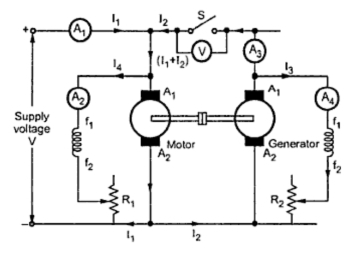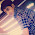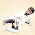Hopkinson's Test

This test is called regenerative test or to back test which can be carried out on two identical d.c. machines mechanically coupled to each other and simultaneously tested. Thus the full load test can be carried out on two identical shunt machines without wasting their outputs. One of the machines is made to act as a motor while the other as a generator. The mechanical output obtained from the motor drives the generator whose electrical output supplies the greater part of input to the motor. The motor is connected to the supply mains only to components for losses since in absence of losses, the motor-generator set would have run without any external power supply. But due to losses, the generator output is not sufficient to drive the motor. Thus motor takes current from the supply to account for losses.
The Fig. 1 shows the connection diagram for Hopikinson's test. The two shunt machines are connected in parallel. One of the machines is then started as a motor. Here the stator connections are not shown for simplicity.Fig. Hopkinson's test

The switch S is kept open. The other machine which is coupled to first will act as load on first which is acting as motor. Thus second machine will act as a generator. The speed of motor is adjusted to normal value with the help of the field rheostat. The voltmeter reading is observed. The voltage of the generator is adjusted by its field rheostat so that voltmeter reading is zero. This will indicate that the generator voltage is having same magnitude and polarity of that of supply voltage. This will prevent heavy circulating current flowing in the local loop of armatures on closing the switch. Now switch S is closed. The two machines can be put into any load by adjusting their field rheostats. The generator current I2 can be adjusted to any value by increasing the excitation of generator or by reducing the excitation of motor. The various reading shown by different ammeters are noted for further calculations.
The input to the motor is nothing but the output of the generator and small power taken from supply. The mechanical output given by motor after supplying losses will in turn drive the generator.
Let        V = Supply VOLTAGER
I1 = Current taken from the supply
I2 = Current supplied by generator
I3 = Exciting current of generator
I4 = Exciting current of motor
Ra = Resistance of armature of each machine
η = Efficiency of both generator and motor.
Input to the motor = V (I1 + I2 )
Output of motor = η x input = V (I1 +I2 )
The output of motor will be given as input to the generator.
Input to generator = η V (I1 +I2 )
Output of generator = η x input = η .η V (I1 + I2 )
= η2 V (I1 + I2 )               ...............  (I)
The output of generator can also be given as,
Output of generator = V . I2                           .......... (II)
From equations (i) and (ii),
η2 V (I1 + I2 )  = I

But the assumption of equal efficiencies of two machines is true in case of only large output machines where difference in armature currents of two machines is not large. Also the difference in excitation current required to circulate full load current in the armature will not effect the iron losses. But in case of small machines the difference between armature and field currents is large. So efficiencies can not be assumed to be same. Here the stray losses are assumed to be equal whereas armature and field copper losses are separately determined for estimating the efficiencies separately.
Armature copper loss in generator = (I2 + I3 )2 Ra
Armature copper loss in motor = (I1 + I2 - I4 )2
Copper loss in field winding of motor = V I3
Copper loss in field winding of motor = V I4
But total losses in generator and motor are equal to the power supplied by the mains.
Power drawn from supply =  V I1
The stary loss for both the machines = V I2 - {{I2 + I3 )2  Ra + (I1 + I2 - I4 )2 Ra +  V I3 + V I4 }
= Ws (say)
Assuming that stray losses are equally divided between the two machines.
Stray loss for each machine = Ws /2
The machine which is acting as a motor
Total losses = (I1 + I2 - I4 )2 Ra + V I4 + Ws /2
Input to motor = (I1 + I2 )
Efficiency of motor, ηm = Output/Input = (Input -  Losses)/Input

The machine which is acting as a generator,
Total losses = (I2 + I3)2  Ra + V I3 + Ws /2
Output of generator = V I2
Efficiency of generator, ηg = Output/Input = Output/(Output + Losses)

The various advantages of Hopkinson's test are,
1. The power required for conducting the test is small compared to full load powers of the two machines.
2. Since the machines are operated at full load conditions, change in iron loss due to distortion in flux at full load will be included in the calculations.
3. As the machines are tested under full load conditions, the temperature rise and quality of communication of the two machines can be observed.
4. The test is economical as power required to conduct the test is very small which is just sufficient to meet the losses.
5. There is no need for arranging any actual load. Similarly by changing the field currents of two machines, the load can be easily changed and a load test over complete range of load can be taken.

The various disadvantages of Hopkinson's test are,
1. There is difficulty in availability of two tdentical machines.
2. The iron losses in the two machines can not be separated. The iron losses are different in both the machines because of different excitations.
3. The machines are not loaded equally in case of small machines which may lead to difficulty in analysis.
This test is better suited in case of large machines.

See solved example on Hopkinson's test

Related Articles :
Ratings of a D.C. Motor

1.here the power drawn from the power supply is v x i1 so please correct the mistake

2.thanks, it have been corrected

3.Nice to see this post .

For Electrical Engineering Information and Interview Questions

visit

Electrical Engineering Info

Like my fb page https://www.facebook.com/electricalengineeringinfo